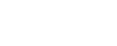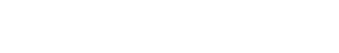1. ## Introduction:

Our group had done 3 data sets for the same experiment process, and had recorded 3 groups of data. The aim of experiments was to determine thepercentage of water in hydrated Ca(NO3)2. We obtained the percentages through experimental procedures of reacting Ca(NO3)2 with Na2CO3 , filtrating and drying CaCO3.

The chemical equation of the reaction is:

Ca(NO3)2 (aq)+Na2CO3 (aq) →2NaNO3 (aq)+CaCO3 (s)

1. ## The Table of Raw Data

2.1 Quantitative Data2.2 Qualitative Data

①All of the solid Ca(NO3)2•4H2O dissolved in water

②White precipitates formed when aqueous Na2CO3 was mixed with Ca(NO3)2 in water.

③After the filtration on Buchner Funnel, white precipitate appeared on the filter paper.

1. ## Data Processing

Use group1 as example.

The mass of CaCO3 could be found by subtracting the mass of filter paper from “0.822g0.001”, then it could be used to calculate the mass of Ca(NO3)2 via reaction equation. mH2O in the hydrate equals m Ca(NO3)2▪4H2O－m Ca(NO3)2.

With the m H2O and mCaCO3, the percentage becomes able to worked out.

The mass of precipitate(CaCO3)=Mass of precipitate and dry filter paper－ Mass of dry filter paper=0.822g-0.300g=(0.822－0.300) = 0.522g

Uncertainty=0.001+0.001=0.200g

∴m(CaCO3)=0.522g±0.002g

The reaction equation: Na2CO3 (aq)+Ca(NO3)2 (aq)→CaCO3(s)+2NaNO3

mCaCO3=0.522g0.002 (from A.),

abs uncertainty=measurement x percentage uncertainty

measurement =0.00522mol ; percentage uncertainty==0.004

abs uncertainty=0.004×0.00522=0.00002mol

∴n CaCO3=0.00522mol±0.00002mol

∵n Ca(NO3)2= n CaCO3 (from the equation)

∴n Ca(NO3)2=0.00522mol±0.00002mol

1. m Ca(NO3)2 =(0.00522mol±0.00002mol)×164.09g/mol

=0.00522mol×164.09 g/mol

=0.857g

abs uncertainty=measurement

abs uncertainty=0.004×0.857=0.003g

∴m Ca(NO3)2=0.857g±0.003g

1. mH2O=m(hydrated Ca(NO3)2﹣m Ca(NO3)2

= 1.507±0.001－0.857±0.003g

= 0.650g±0.004gI.e., according to the 1st experiment, water content in the hydrate is 43.1%

Three times of experiment showed 3 distinct results:

0.431±0.003(1st ), 0.364±0.004 (2nd), 0.322±0.0089 (3rd)

Average Value=(0.431+0.364+0.322) ÷3=0.372g

Half Range=(max value-mini value) ÷2=(0.431﹣0.322) ÷2=0.0545

∵half range＞abs uncertainties of all the measurements,∴Result=Average value±half range=0.372g±0.0545g

1. ## Conclusion

1st experiment

% uncertainty=(0.003/0.431)*100%=0.007=0.7%

% error was much larger than % uncertainty, so systematic error was more significant, the deviation were caused by improper operation.

2nd experiment

% uncertainty=(0.004/0.364)*100%=0.01=1%

% error was still larger than % uncertainty, systematic error was more significant. but the % error was much smaller than the 1st one, means that operation improves.

3rd experiment

% uncertainty=(0.0089/0.322)*100%=0.028=2.8%

Percentage error was much smaller than previous experiments.

1. ## Evaluation

(systematic)Not all the precipitate was transformed from beaker to funnels during filtration process.

(systematic)Some precipitate was leaked for the filter paper that was not closely stuck to funnel.

(systematic) Some precipitate dropped before stoving

The influence of systematic errors①→③: The loss of precipitate resulted in smaller weight of CaCO3. In the chemical equation, the ratio of CaCO3 to Ca(NO3)2 is 1:1, so the mass of Ca(NO3)2 decreases as the mass of CaCO3 decreases. The mass of Ca(NO3)2 subtracted from the hydrate became smaller, so the proportion of water in the hydrate became bigger, thus the percentage of water is larger than expected value.

(systematic) The primary error among the process was that some Ca(NO3)2 solution was lost during the 1st experiment. Some liquid dropped out of the beaker by accident, the amount (mol) of the reactant Ca(NO3)2 was reduced significantly. So that the result of the 1st group was much larger than theoretical value.

(random) The experiment was only repeated 3 times, the precision of data was limited.

1. ## Improvements

①When tearing the filter paper to fit it in the funnel, we should prevent it being too small, and we’d better apply more water along the edge to stick it more tightly.

②When measuring solution, we should keep our sight and the concave meniscus at the same level.

③It would be better to use distilled water to clean the beaker after each single time of experiment

④When taking out the glass dish from the oven, we should make sure the filter paper and the precipitate is totally dried.

Cite this article as: William Anderson (Schoolworkhelper Editorial Team), "Lab Report: Determine The Percentage Of Water In Hydrated Calcium nitrate," in SchoolWorkHelper, 2019, https://schoolworkhelper.net/lab-report-determine-the-percentage-of-water-in-hydrated-calcium-nitrate/.